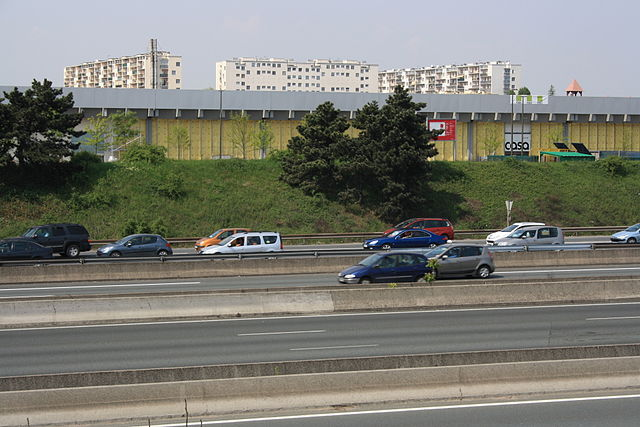An algebra problem by A Former Brilliant Member

Algebra Level 1Artik and Bide are positioned in two cars that are 100 kilometers apart on a straight road. If they both travel in the same direction, then they will meet in 5 hours. If however, they travel towards each other, then they will meet in 1 hour. Let $u$ and $v$ be the speeds (in km/hr) of Artik's and Bide's car respectively. Find $\frac{u-v}{2}.$

Note: $u > v$

×